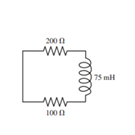# Problem: At t = 0 s, the current in the circuit in the figure is I0. At what time is the current ½ I0? Express your answer with the appropriate units.

###### FREE Expert Solution

Equivalent resistance for resistors in series:

$\overline{){{\mathbf{R}}}_{{\mathbf{eq}}}{\mathbf{=}}{{\mathbf{R}}}_{{\mathbf{1}}}{\mathbf{+}}{{\mathbf{R}}}_{{\mathbf{2}}}{\mathbf{+}}{\mathbf{.}}{\mathbf{.}}{\mathbf{.}}{\mathbf{+}}{{\mathbf{R}}}_{{\mathbf{n}}}}$

For an LR circuit, the variation of current as a function of time is:

$\overline{){\mathbf{I}}{\mathbf{\left(}}{\mathbf{t}}{\mathbf{\right)}}{\mathbf{=}}{{\mathbf{I}}}_{{\mathbf{0}}}{{\mathbf{e}}}^{\mathbf{-}\mathbf{t}}{\mathbf{z}}}}$

Equivalent resistance in the circuit:

Req = 200 + 100 = 300Ω

82% (487 ratings)###### Problem Details

At t = 0 s, the current in the circuit in the figure is I0. At what time is the current ½ I0? Express your answer with the appropriate units.